# Deriving Gauss’s Law from Coulomb’s Law

In the world of classical electromagnetism, we can understand the interaction between electricity and magnetism through four fundamental equations, known as Maxwell’s equations. Derived originally by James Clerk Maxwell in the 19th century, in his infamous paper “On Physical Lines of Force” as a response to all of Michael Faraday’s empirical observations about electromagnetism, these equations form the basis of modern telecommunications, electric circuits, and more. Deriving Maxwell’s equations is no easy feat, but it can be an incredibly rewarding exercise for students of physics and electrical engineering alike, or just about anyone who loves to see mathematics in action. In this short article, I will present a mathematical way of deriving one of these equations, known also as “Gauss’s law of electric fields”, written mathematically as

and what it tells us about electric fields.

We shall begin our derivation by making use of Coulomb’s law. Let us consider two electric charges in space, namely q1 and q2. It is known from empirical observation that the electrostatic force between such charges is inversely proportional to the square of the distance between them, and directly proportional to the product of the two charges. We can write this as Eq(1) below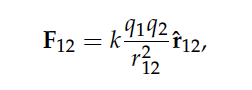Equation 1

where k is a constant related to the permittivity of the medium via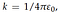Equation 2

The vector r12 is in fact the difference between the displacement vectors of charges 1 and 2 respectively, or simply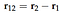Equation 3

Using the definition of the unit vector as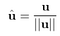Equation 4

We now write the Coulomb force term as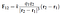Equation 5

Now, from Newton’s 3rd law, we know that forces have equal and opposite reactions, therefore we can write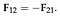Equation 6

Furthermore, the force exerted on an electric charge q due to an electric field E is defined as F = qE. Therefore we express the electric field as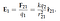Equation 7

What does this actually mean? It means that What does this actually mean? It means that the electric field E1 due to charge q2 unto a charge q1 is dependent only on the magnitude of q2. The notation might seem confusing, but we can remember this because the field should always be point outwards from a positive charge, and thus if we measure the field around a positive charge q2, then the field lines will be directed along the vector r12(that is, pointing from q2 to q1). A lot of textbooks like to drop this notation altogether and write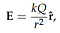Equation 8

although caution must be taken to avoid confusion. Because the electric field lines from a positive point charge Q are directed radially outwards (and inwards for a negative charge), the field possesses perfect spherical symmetry. By Newton’s second law (a.k.a F = ma), we know the net force acting on a body is the sum total of all the forces acting on it, and in the context of electric charges we can easily write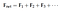Equation 9

By the same reasoning, we write the total electric field due to all the electric charges in some arbitrary space as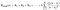Equation 10

Thus in the absence of any other forces, the electric field is always oriented along the direction of the force. This rather trivial result leads to an all more important one: if we replace the discrete space of charges q with a continuum of charge, known as the charge density 𝝆(r) in units of C/m^3 (note that charge is a discrete quantity, but for large amounts we can approximate the sum by an integral) we write the integral over all coordinates r’ away from the position r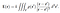Equation 11

It is therefore possible to calculate the electric field due to any charge distribution, provided we know what the charge density function 𝝆(r) is.

Now that we have derived a general expression for the electric field E(r) as a function of a continuous density of charge 𝝆(r), we can start to think about what happens when we perform mathematical operations on E(r). Let us for instance, take the divergence of the field in Eq(11)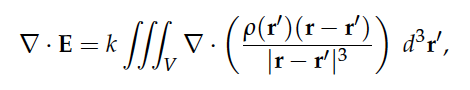Equation 12

the divergence operator on the right-hand side of the equation can be freely placed inside or outside of the triple-integral, because both the divergence and integral operators are linear (e.g. if you think of the integral as a sum, then the divergence of the sum of a bunch of functions is the same as the sum of the divergence of that same bunch of functions). We note that since the divergence operator acts with respect to r rather than r’, the function 𝝆(r’) can be moved outside of the divergence operator, namely we rewrite the equation as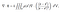Equation 13

Now all that remains for us to solve this problem is to know what the divergence term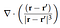Equation 14

is simplified to. To do this, we will invoke the divergence theorem, namely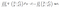Equation 15

which basically means that the volume integral of the divergence of some function is the same as the surface integral of that function oriented along a set of normal vectors n that are always pointing perpendicular to the surface element dS. If we now suppose that we choose a sphere of radius R, it follows that the unit normal vector to the surface will always be directed radially outwards from the sphere, allowing us to write

additionally |r - r’| = R. Now, the surface area element in spherical coordinates is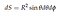Equation 16

which means that our divergence integral reduces to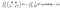Equation 17

This rather surprising result has a very peculiar implication: the divergence term in Eq(14) must be equal to some function which upon being integrated is equal to a constant of 4𝛑. One such function with this kind of property is the Dirac-delta function (or distribution for those more mathematically inclined), namely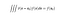Equation 18

Thus, this suggests we can defineEquation 19

Leading to the result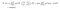Equation 20

and so we now obtain the desired equation for Gauss’s law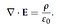Equation 21: Gauss’s law in differential form

In differential form, this equation tells us that the amount of field E passing through an infinitesimally small volume of space (let us denote it as dV) is equal to the charge density in that localised region, divided by the permittivity of free space. A better insight is gained by looking at it in integral form: let us integrate both sides with respect to a volume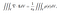Equation 22

the integral on the right hand side is nothing more than the total charge Q, enclosed in the volume V. Then, upon using the divergence theorem we turn the left-hand side into a surface integral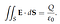Equation 23: Gauss’s law in integral form

Here, the total flux of the field E is equal to the total amount of charge enclosed by a surface S. To prove this statement, we shall consider the electric field due to a point charge Q centred at the origin, whose field in three dimensions is oriented radially outwards in spherical coordinates (this is the expression we derived previously using Coulomb’s law)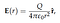Equation 24

and choosing a spherical surface of fixed radius R centred at the origin, with the same surface element as before oriented radially outwards

we obtain

as required. The most important consequence of this law is that it does not matter where we place our surface S around the charge Q, the electric flux will always be the same, even if the field lines are not aligned with the surface normal vectors. Therefore, the electric flux through an arbitrary surface S is only dependent on the enclosed charge Q. As an example, suppose we had a discrete distribution of charges in space such that Q = Sum of all q. Then, the total electric flux out of an arbitrary closed surface S surrounding them is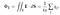Equation 25: electric flux

it should be noted also that negative charges inside the surface remove flux. Consider a simple dipole made of two equal and opposite charges q and -q. For any arbitrary surface surrounding them, it is easy to show that the net electric flux is zero

However, if we compute the total electric flux surrounding each of the charges by placing two disjoint surfaces around them, we see that the fluxes will be equal and opposite, namely

Hence, the total flux in a region Ω composed of n sub-regions, all enclosed inside Ω is nothing more than the sum of all individual fluxes inside the region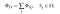Equation 26

This concludes Gauss’s law of electric fields.

Oscar is a physicist, educator and STEM enthusiast. He is currently finishing a PhD in Theoretical Physics with a focus on photonics and stochastic dynamics.